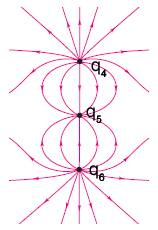# Point Charge Given Field Maps

## Homework Statement

The electric field map is shown below. (Take it to be a self-consistent scale drawing at a particular moment in time.)where $\mathrm{q}_4 = +10 C, \mathrm{q}_6 = +10 C$

What is the charge of $\mathrm{q}_5$ ?

## The Attempt at a Solution

I've tried the following values for $\mathrm{q}_5$:

$$-5,-10,-15,-20$$

and none of them are correct according to the online homework software. How would I find the charge of $\mathrm{q}_5$?

Thank you,

BvU
Homework Helper
It looks as if halfway q4 and q5 the field is parallel to the axis at small distances x from the axis.
Write down the three contributions ##\perp## to the axis and make sure they cancel to first order in x.

And I wonder if three seemingly random shots at the answer counts as an attempt at solution :)
(If you have unlimited tries, step from -10 to -20 in very small steps ! ;) )

DEvens
Gold Member
What do you suppose it means that the drawing is "a self-consistent scale drawing"? What will that mean with regard to the field lines?

It's not too often that a physics problem can be solved by a Sesame Street character. "Ten! Ten field lines! Heh! Heh! Heh!"

•BvU
BvU
You were right; each field line represents an equal fraction of the original charge. The answer came out to around $$-7.1 \mathrm{C}$$.
•